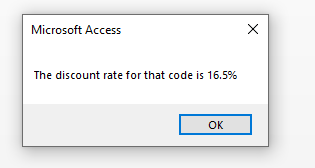# Controlling Program Flow

Controlling program flow in VBA or in any other language is directing program activity based on the user’s
input, result of some logic, or actions that are performed.

You can selectively perform tasks, and respond to different situations.

To this end, I will be demonstrating the following 2 control structures:

1. If Structures
-If…Then
-If…Then…Else
-If…Then…ElseIf…Else

2. Select Case Structure
-This is like the “Switch” structure in other languages

In my opinion, I think the Select Case Structure is easier to read and follow.

## If Statement In VBA

The If statements (control structures) start with an If…Then and close with an End If

The If statement results analyzes a True or False condition.

## The Single line If…Then statement:

If blnTall = True Then MsgBox “Tall”

## The If…Then…End If statement (1 possible outcome):

The pseudocode for this is:

If condition Then
statements
End if

Another example includes a variable assignment:

```If strPassword = "h3j2" Then
MsgBox "Welcome To The Portal"
End If

```

## The If…Then…Else…End If statement (2 possible outcomes):

The pseudocode for this is:

If condition Then
statements
Else
statements
End if

Msgbox “Welcome”
Else
End if

## The If…Then…ElseIf…Else…End If statement (3+ possible outcomes):

If you need to check for three or more alternatives, you can use this structure. I would most likely try to use a Select Case structure for 3 or more alternatives, but if you are forced into using this and not the Select Case (or you are using Python, which has no Switch statement!), here it is:

The pseudocode for this is:

If condition Then
statements
ElseIf condition
statements
Else
statements
End if

```If strShipState = "Hawaii" Then
dblShipCost = 15
dblShipCost = 10
Else
dblShipCost = 5
End If
```

## Select Case In VBA

The Select Case structure in VBA is a great choice when you have to evaluate mutiple variable scenarios.

The pseudocode for this is:

Select Case expression
Case what-expression-is1
statements
Case what-expression-is2
statements
Case what-expression-is3
statements
Case Else
statements
End Select

The Select Case structure is efficient because it evaluates the test expression only once.

The result of the expression is then compared against mutiple values to determine which code block is invoked.

Consider the following which determines a salesperson’s bonus base on the amount of an salesperson’s sales :

If their sales totals are under \$10,000 they’ll only get a 10% bonus,
but if they are above \$10,000 but below \$20,000 they’ll get a 15% bonus.

Otherwise, they’ll get the normal 25% bonus.

```Select Case dblSales
Case Is < 10000
intBonus = 0.1 * dblSales
Case Is < 20000
intBonus = 0.15 * dblSales
Case Else
intBonus = 0.25 * dblSales
End Select
```

...and finally using this Select Case structure, evaluates the amount of discount that should be applied based on the code:

```Sub FindDiscount()
Dim strCode As String
Dim dblDiscount As Double 'Double because the discount may have decimals
'Get the discount code from the user
strCode = InputBox("Enter discount code")
'Get the discount amount based on the code
Select Case strCode
Case "B1"
dblDiscount = 34.5
Case "B2", "C2"
dblDiscount = 16.5
Case Else
dblDiscount = 0
End Select
'Display the discount rate to the user
MsgBox "The discount rate for that code is " & dblDiscount & "%"
End Sub
```

When you run it, you should get:This was part of Free Access programming tutorial Video 1

Need help, let me know.

#### “Go Fund Me” Page(\$5 suggested amount)## Free! Subscribe To Our YouTube Channel!